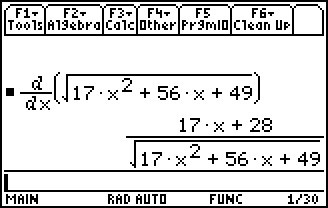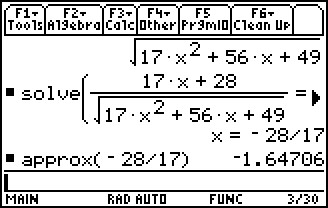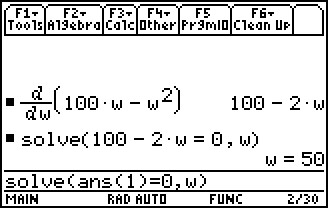# Activities

•##### Download

•• ##### Subject Area

• Math: Calculus: Applications of the Derivative

• ##### Author9-12

45 Days

• ##### Device
• TI-89 / TI-89 Titanium
• ##### Report an Issue

Optimization#### Activity Overview

Students will learn how to use the second derivative test to find maxima and minima in word problems and solve optimization in parametric functions.

#### Key Steps

•In Problem 1, students investigate optimization of distance and area problems. They begin by graphing a linear equation and considering a point (x, y) on the line. Students seek to find the coordinates of the point that minimizes distance from the point to the origin.

Here, the linear equation acts as the constraint, which students may use to rewrite the distance function in one variable.

•Students then find the first derivative, and apply the second derivative test to find the minimum at the critical value of x. They substitute the value of x into the original linear equation to determine the y-coordinate.

•Also in Problem 1, students will consider a rectangle with a fixed perimeter of 200 m, seeking to maximize its area. In a manner similar to that above, students maximize the function A = l w with the constraint 2l + 2w = 200. They can confirm their answer by using the fmax command to obtain value of w.